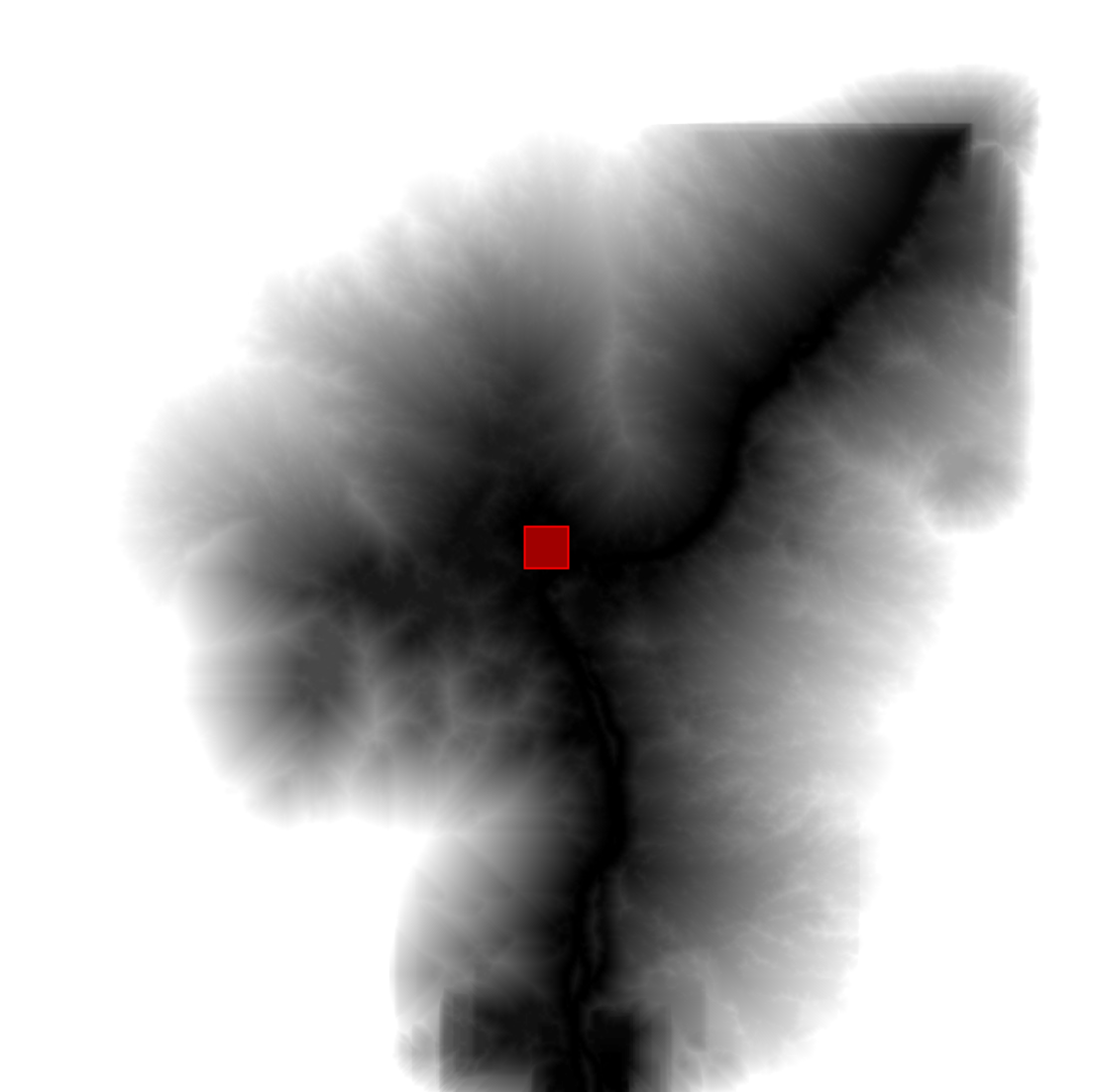# Cumulative Cost Mapping

Use `image.cumulativeCost()` to compute a cost map where every pixel contains the total cost of the lowest cost path to the nearest source location. This process is useful in a variety of contexts such as habitat analysis (Adriaensen et al. 2003), watershed delineation (Melles et al. 2011) and image segmentation (Falcao et al. 2004). Call the cumulative cost function on an image in which each pixel represents the cost per meter to traverse it. Paths are computed through any of a pixel's eight neighbors. Required inputs include a `source` image, in which each non-zero pixel represents a potential source (or start of a path), and a `maxDistance` (in meters) over which to compute paths. The algorithm finds the cumulative cost of all paths less than maxPixels = `maxDistance`/scale in length, where scale is the pixel resolution, or scale of analysis in Earth Engine.

The following example demonstrates computing least-cost paths across a land cover image:

```// A rectangle representing Bangui, Central African Republic.
var geometry = ee.Geometry.Rectangle([18.5229, 4.3491, 18.5833, 4.4066]);

// Create a source image where the geometry is 1, everything else is 0.
var sources = ee.Image().toByte().paint(geometry, 1);

// Mask the sources image with itself.
sources = sources.updateMask(sources);

// The cost data is generated from classes in ESA/GLOBCOVER.
var cover = ee.Image('ESA/GLOBCOVER_L4_200901_200912_V2_3').select(0);

// Classes 60, 80, 110, 140 have cost 1.
// Classes 40, 90, 120, 130, 170 have cost 2.
// Classes 50, 70, 150, 160 have cost 3.
var cost =
cover.eq(60).or(cover.eq(80)).or(cover.eq(110)).or(cover.eq(140))
.multiply(1).add(
cover.eq(40).or(cover.eq(90)).or(cover.eq(120)).or(cover.eq(130))
.or(cover.eq(170))
.multiply(2).add(
cover.eq(50).or(cover.eq(70)).or(cover.eq(150)).or(cover.eq(160))
.multiply(3)));

// Compute the cumulative cost to traverse the land cover.
var cumulativeCost = cost.cumulativeCost({
source: sources,
maxDistance: 80 * 1000 // 80 kilometers
});

// Display the results
Map.setCenter(18.71, 4.2, 9);
Map.addLayer(cover, {}, 'Globcover');
Map.addLayer(cumulativeCost, {min: 0, max: 5e4}, 'accumulated cost');
Map.addLayer(geometry, {color: 'FF0000'}, 'source geometry');
```

The result should look something like Figure 1, in which each output pixel represents the accumulated cost to the nearest source. Note that discontinuities can appear in places where the least cost path to the nearest source exceeds maxPixels in length.Figure 1. The cumulative cost to the source pixels, where cost is determined by the land cover categories. Low costs are black, higher costs are white.

Текущей странице